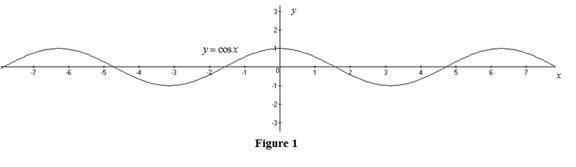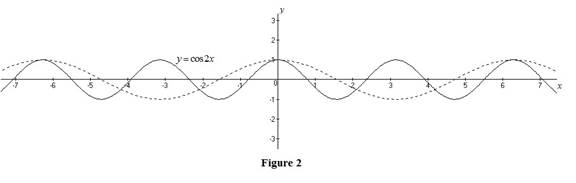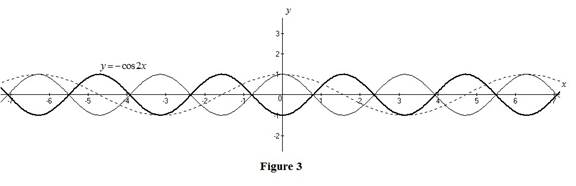# The graph of the function y = − cos 2 x by using transformation.### Single Variable Calculus: Concepts...

4th Edition
James Stewart
Publisher: Cengage Learning
ISBN: 9781337687805### Single Variable Calculus: Concepts...

4th Edition
James Stewart
Publisher: Cengage Learning
ISBN: 9781337687805

#### Solutions

Chapter 1, Problem 15RE
To determine

## To sketch: The graph of the function y=−cos2x by using transformation.

Expert Solution

### Explanation of Solution

Graph:

The standard graph of the function y=cosx is roughly sketched as shown below in Figure 1.Then, to draw the graph of y=cos2x, shrink the graph y=cosx horizontally by a factor of 2. Thus, the graph of y=cos2x is shown below in Figure 2.Then, to draw the graph of y=cos2x, reflect the graph y=cos2x about the x-axis. Thus, the graph of y=cos2x is shown below in Figure 3.Observe that Figure 3 is obtained from Figure 1 in such a way that it is compressed 2 units horizontally and then reflected about the x-axis.

### Have a homework question?

Subscribe to bartleby learn! Ask subject matter experts 30 homework questions each month. Plus, you’ll have access to millions of step-by-step textbook answers!